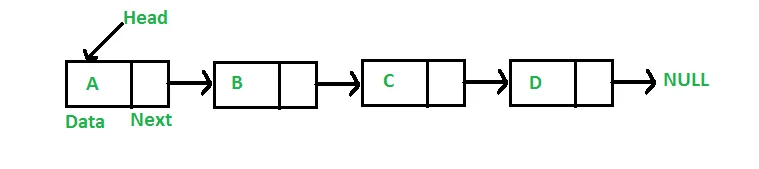## 什么是链表### 链表怎样用代码表示，以及插入、删除、遍历操作#

/// <summary>
/// 链表中的节点结构
/// </summary>
public class Node
{
public int data;
public Node next;

public Node (int data)
{
this.data = data;
}
}

{
public Node tail;

/// <summary>
/// 插入一个节点
/// </summary>
/// <param name="data"></param>
public void InsertNode(int data)
{
Node newNode = new Node(data);
{
tail = newNode;
}
else
{
tail.next = newNode;
tail = newNode;
}
}

/// <summary>
/// 删除一个节点
/// </summary>
/// <param name="node"></param>
public void DeleteNode(Node node)
{
// 只有一个节点的情况
if(head == tail && head == node)
{
tail = null;
}
else
{
Node prev = head;
Node opt = head.next;
while(opt != null)
{
// 如果当前检查的节点就是要删除的节点，
// 则用上一个节点，指向当前操作节点的下一个节点，
// 这样就把当前节点从链表中剔除了。
if(node == opt)
{
prev.next = opt.next;
break;
}
else
{
prev = opt;
opt = opt.next;
}
}
}
}

/// <summary>
/// 遍历整个链表，调试打印数据
/// </summary>
public void LoopDebug()
{
Node opt = head;
while(opt != null)
{
Debug.LogFormat("Data: {0}", opt.data);
opt = opt.next;
}
}
}


## 再深入一点点#

/// <summary>
/// 双向链表中的节点结构
/// </summary>
public class Node
{
public int data;
public Node next;
public Node prev;

public Node (int data)
{
this.data = data;
}
}


注意和添加和删除函数中的代码变化

public class Linkedlist
{
public Node tail;

/// <summary>
/// 插入一个节点
/// </summary>
/// <param name="data"></param>
public void InsertNode(int data)
{
Node newNode = new Node(data);
{
tail = newNode;
}
else
{
newNode.prev = tail;
tail.next = newNode;
tail = newNode;
}
}

/// <summary>
/// 删除一个节点
/// </summary>
/// <param name="node"></param>
public void DeleteNode(Node node)
{
// 只有一个节点的情况
if(head == tail && head == node)
{
tail = null;
}
else
{
Node prevNode = head;
Node optNode = head.next;
while(optNode != null)
{
if(node == optNode)
{
if(optNode.next != null)
{
optNode.next.prev = prevNode;
}
prevNode.next = optNode.next;
break;
}
else
{
prevNode = optNode;
optNode = optNode.next;
}
}
}
}

/// <summary>
/// 遍历整个链表，调试打印数据
/// </summary>
public void LoopDebug()
{
Node opt = head;
while(opt != null)
{
Debug.LogFormat("Data: {0}", opt.data);
opt = opt.next;
}
}
}